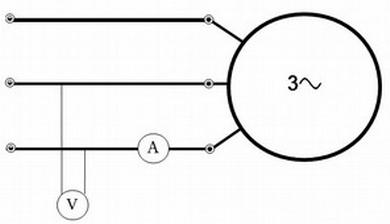# kVA calculator. Apparent power

## Calculator

 Single phase Three phase Voltage: V (Volt) kV (kilo-volt) Current: Parametro3*Ud3/Parametro2/Ud2Parametro3*Ud3/Parametro2/Ud2 A (Amp) kA (kilo-amp) Apparent power: VA kVA

## Theory

### Apparent power (S)

, in kVA (kilovolt-amps), is a measure of the power in an electrical circuit. Although is one of the most relevant parameters for many electrical equipment, it is not the real power. To calculate the real power the apparent power must be multiplied by the power factor, which is always less than or equal to one. So, when power factor is 1 (e.g. for an electrical resistor) then kVA = kW. You can see a more detailed explanation in the following link: Power factor calculator

### Calculation for single-phase circuits

It is calculated by multiplying the voltage (V) by the current (I).
S (VA) = I(A) × V(V)
S (kVA) = I(A) × V(V) / 1000

### Calculation for three-phase circuits

. In this case it is very similar but we have to multiply by the square root of 3 :
S(VA) = √3 × I(A) × V (V)
S(kVA) = √3 × I(A) × V (V) / 1000